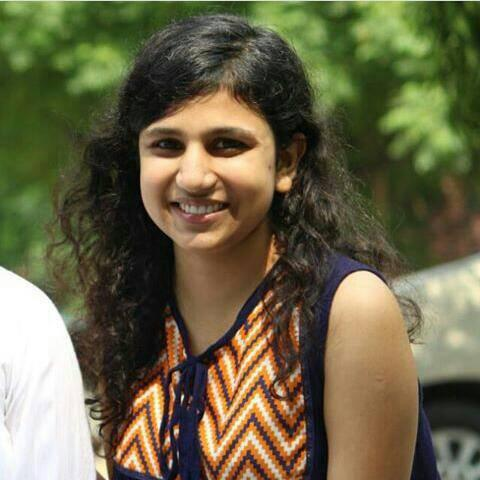# Largest and smallest digit of a number

Given a number N. The task is to find the largest and the smallest digit of the number.

Examples :

Input : N = 2346
Output : 6 2
6 is the largest digit and 2 is samllest

Input : N = 5
Output : 5 5

## Recommended: Please try your approach on {IDE} first, before moving on to the solution.

Approach: An efficient approach is to find all digits in the given number and find the largest and the smallest digit.

## C++

 `// CPP program to largest and smallest digit of a number ` `#include ` `using` `namespace` `std; ` ` `  `// Function to the largest and smallest digit of a number ` `void` `Digits(``int` `n) ` `{ ` `    ``int` `largest = 0; ` `    ``int` `smallest = 9; ` ` `  `    ``while` `(n) { ` `        ``int` `r = n % 10; ` ` `  `        ``// Find the largest digit ` `        ``largest = max(r, largest); ` ` `  `        ``// Find the smallest digit ` `        ``smallest = min(r, smallest); ` ` `  `        ``n = n / 10; ` `    ``} ` `    ``cout << largest << ``" "` `<< smallest; ` `} ` ` `  `// Driver code ` `int` `main() ` `{ ` `    ``int` `n = 2346; ` ` `  `    ``// Function call ` `    ``Digits(n); ` ` `  `    ``return` `0; ` `} `

## Java

 `// Java program to largest and smallest digit of a number ` `import` `java.util.*; ` `import` `java.lang.*; ` `import` `java.io.*; ` ` `  `class` `Gfg ` `{ ` `     `  `// Function to the largest and smallest digit of a number ` `static` `void` `Digits(``int` `n) ` `{ ` `    ``int` `largest = ``0``; ` `    ``int` `smallest = ``9``; ` ` `  `    ``while``(n != ``0``)  ` `    ``{ ` `        ``int` `r = n % ``10``; ` ` `  `        ``// Find the largest digit ` `        ``largest = Math.max(r, largest); ` ` `  `        ``// Find the smallest digit ` `        ``smallest = Math.min(r, smallest); ` ` `  `        ``n = n / ``10``; ` `    ``} ` `    ``System.out.println(largest + ``" "` `+ smallest); ` `} ` ` `  `// Driver code ` `public` `static` `void` `main (String[] args) ``throws` `java.lang.Exception ` `{ ` `    ``int` `n = ``2346``; ` ` `  `    ``// Function call ` `    ``Digits(n); ` ` `  `} ` `} ` ` `  `// This code is contributed by nidhiva `

## Python3

 `# Python3 program to largest and smallest digit of a number ` ` `  `# Function to the largest and smallest digit of a number ` `def` `Digits(n): ` `    ``largest ``=` `0` `    ``smallest ``=` `9` ` `  `    ``while` `(n): ` `        ``r ``=` `n ``%` `10` ` `  `        ``# Find the largest digit ` `        ``largest ``=` `max``(r, largest) ` ` `  `        ``# Find the smallest digit ` `        ``smallest ``=` `min``(r, smallest) ` ` `  `        ``n ``=` `n ``/``/` `10` ` `  `    ``print``(largest,smallest) ` ` `  ` `  `# Driver code ` ` `  `n ``=` `2346` ` `  `# Function call ` `Digits(n) ` ` `  `# This code is contributed by mohit kumar 29 `

## C#

 `// C# program to largest and ` `// smallest digit of a number ` `using` `System; ` `     `  `class` `GFG ` `{ ` `     `  `// Function to the largest and  ` `// smallest digit of a number ` `static` `void` `Digits(``int` `n) ` `{ ` `    ``int` `largest = 0; ` `    ``int` `smallest = 9; ` ` `  `    ``while``(n != 0)  ` `    ``{ ` `        ``int` `r = n % 10; ` ` `  `        ``// Find the largest digit ` `        ``largest = Math.Max(r, largest); ` ` `  `        ``// Find the smallest digit ` `        ``smallest = Math.Min(r, smallest); ` ` `  `        ``n = n / 10; ` `    ``} ` `    ``Console.WriteLine(largest + ``" "` `+ smallest); ` `} ` ` `  `// Driver code ` `public` `static` `void` `Main (String[] args) ` `{ ` `    ``int` `n = 2346; ` ` `  `    ``// Function call ` `    ``Digits(n); ` `} ` `} ` ` `  `// This code is contributed by PrinciRaj1992  `

Output:

```6 2
```

Don’t stop now and take your learning to the next level. Learn all the important concepts of Data Structures and Algorithms with the help of the most trusted course: DSA Self Paced. Become industry ready at a student-friendly price.

My Personal Notes arrow_drop_upCheck out this Author's contributed articles.

If you like GeeksforGeeks and would like to contribute, you can also write an article using contribute.geeksforgeeks.org or mail your article to contribute@geeksforgeeks.org. See your article appearing on the GeeksforGeeks main page and help other Geeks.

Please Improve this article if you find anything incorrect by clicking on the "Improve Article" button below.

Article Tags :
Practice Tags :

1

Please write to us at contribute@geeksforgeeks.org to report any issue with the above content.